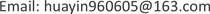﻿ 政府R & D资助对企业自主科技创新的影响——基于门槛回归分析 Influence of Government R & D Funding on Independent Scientific and Technological Innovation of Enterprises—Based on Threshold Regression Analysis

Vol. 08  No. 07 ( 2019 ), Article ID: 31199 , 6 pages
10.12677/ASS.2019.87155

Influence of Government R & D Funding on Independent Scientific and Technological Innovation of Enterprises

—Based on Threshold Regression Analysis

Yin Hua, Xiang Yuan

School of Economics and Management, Shanghai Maritime University, ShanghaiReceived: Jun. 18th, 2019; accepted: Jul. 2nd, 2019; published: Jul. 9th, 2019ABSTRACT

This paper uses the fixed effects model and threshold regression method developed by Hansen to make an empirical analysis of the panel data of 38 industries of industrial enterprises above the scale in China from 2012 to 2017. The research results show that, at present, Chinese government R & D funding has a significant positive impact on enterprise scientific and technological innovation, and the impact will show an “inverted u-shaped” distribution with the increase of government funding.

Keywords:Government R & D Funding, Threshold Regression, Fixed Effects Model, Enterprise Technological Innovation

——基于门槛回归分析8日；录用日期：2019年7月2日；发布日期：2019年7月9日1. 引言

1912年，熊·彼得  在其著作《经济发展理论》一书中，提出了创新理论这一独特的经济理论，人们越发的关注起了技术创新对于经济增长到底起到了怎么样的作用。我国期望在2020年建设成为创新型国家，因此，对于政府R & D资助的大中型企业的创新的影响，必定要将其置于转型经济中科技创新改革的制度背景之下  。

2. 文献综述

3. 实证分析

3.1. 模型设定与变量说明

${y}_{it}=f\left(K,L\right)=A{K}^{\alpha }{L}^{\beta }{e}^{{\mu }_{i}+{\epsilon }_{it}}$ (1)

${y}_{it}=f\left(K,L\right)=AGO{V}_{it}^{{\alpha }_{1}}{S}_{it}^{{\alpha }_{2}}{F}_{it}^{{\alpha }_{3}}{O}_{it}^{{\alpha }_{4}}{e}^{{u}_{i}+{\epsilon }_{it}}$ (2)

$\mathrm{ln}\left({y}_{it}\right)=\delta +{\alpha }_{1}\mathrm{ln}GO{V}_{it}+{\alpha }_{2}\mathrm{ln}{S}_{it}+{\alpha }_{3}\mathrm{ln}{F}_{it}+{\alpha }_{4}\mathrm{ln}{O}_{it}+\beta \mathrm{ln}{L}_{it}+{\theta }^{\prime }{X}_{it}+{\mu }_{i}+{\epsilon }_{it}$ (3)

3.2. 数据来源

3.3. 实证分析

3.3.1. 政府R & D资助对企业技术创新的有效性检验Table 1. Impact of government R & D funding on corporate technology innovation

3.3.2. 政府R & D资助的门槛分析

$\mathrm{ln}\left({y}_{it}\right)=\delta +\beta \mathrm{ln}{L}_{it}+{\lambda }_{1}\mathrm{ln}GO{V}_{it}×I\left({q}_{it}\le \tau \right)+{\lambda }_{2}\mathrm{ln}GO{V}_{it}×I\left({q}_{it}>\tau \right)+{\theta }^{\prime }{X}_{it}+{\mu }_{i}+{\epsilon }_{it}$ (4)

4. 结论及政策建议

4.1. 结论

4.2. 政策建议

4.3. 不足

Influence of Government R & D Funding on Independent Scientific and Technological Innovation of Enterprises—Based on Threshold Regression Analysis[J]. 社会科学前沿, 2019, 08(07): 1122-1127. https://doi.org/10.12677/ASS.2019.87155

1. 1. 熊•彼得. 经济发展理论[M]. 北京: 商务印书馆, 1990.

2. 2. 张杰, 郑文平, 翟福昕. 竞争如何影响创新: 中国情景的新检验[J]. 中国工业经济, 2014(11): 56-68.

3. 3. 许治. 政府公共R&D与内生经济增长[D]: [博士学位论文]. 西安: 西北大学, 2006.

4. 4. 汤伟, 牛文举, 陈效林. 溢出效应对竞争企业研发投资和政府补贴的影响[J]. 现代经济探讨, 2019(3): 65-70.

5. 5. 黄丹丹. 风险投资、政府补贴对科技型上市公司技术创新的影响研究[D]: [硕士学位论文]. 兰州: 兰州财经大学, 2018.

6. 6. 张晓月, 张鑫. 政府专利奖励提升了中小企业绩效吗?——基于知识产权能力调节作用的实证检验[J]. 科技管理研究, 2019, 39(8): 134-140.

7. 7. Cerqua, A. and Pellegrini, G. (2014) Do Subsidies to Private Capital Boost Firms Growth? A Multiple Regression Discontinuity Design Approach. Journal of Public Economics, 109, 114-126. https://doi.org/10.1016/j.jpubeco.2013.11.005

8. 8. Brandt, L., Van Bieseboreck, J. and Zhang, Y. (2012) Creative Accounting or Creative Destruction? Firm-Level Productivity Growth in Chinese Manufacturing. Journal of Development Economics, 97, 339-351. https://doi.org/10.1016/j.jdeveco.2011.02.002